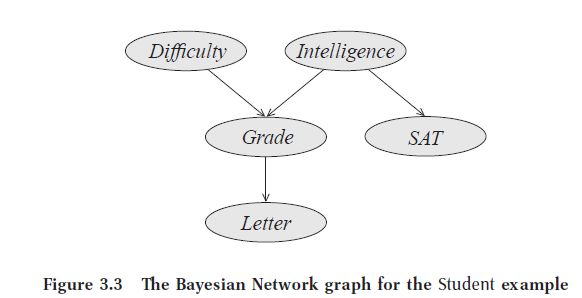Show Question
Math and science::INF ML AI::probabilistic graphical models

# Belief Networks (by Koller)

Koller's approach to Belief networks (which she calls Bayesian Networks) is more intuative than Barber's. Consider the student model:Difficulty: difficulty of a test. Grade: a course grade received by the student. SAT: the student's SAT score. Letter: whether the student's letter of recommendation from the course professor was positive or not. The professor only consults the student's recorded course grade.

Koller proceeds by enumerating the independent assumptions in this model. We can reason that:

1. The professor's recommendation letter depends only on the student's grade in the class.

This can be expressed as:

$(L \perp I, D, S \vert G)$

In other words, once we know the student's grade, our beliefs about the quality of the recommendation letter are not influenced by information about any of the other variables.

2. The student's SAT score depends only on the student's intelligence:

$(S \perp D, G, L \vert I)$
3. Once we know that a student has high intelligence, his SAT score gives no additional information towards predicting his grade:

$(G \perp S \vert I, D)$
4. Intelligence is independent of the test difficulty:

$(I \perp D)$
5. Knowing that a student is smart (or not) does not influence our beliefs in the test difficulty:

$(D \perp I, S)$

Koller describes this whole thing in more detail. She explains how, intuitively, a parent variable "shields" its descendants from probabilistic influence from other variables. Once I know the value of the parents, no information relating directly or indirectly to the parents or other ancestors can influence my beliefs.

With this model in mind, the formal definition follows quite naturally.

### Bayesian network, definition

A Bayesian network structure $$G$$ is a directed acyclic graph whose nodes represent random variables $$X_1, ..., X_n$$. Let $$Pa_{X_i}^G$$ denote the parents of $$X_i$$ in $$G$$, and $$\operatorname{NonDescendants}_{X_i}$$ denote the variables in the graph that are not descendants of $$X_i$$. Then $$G$$ encodes the following set of conditional independence assumptions, called the local independencies, and denoted by $$\mathcal{I}_{l}(G)$$:

$\text{For each variable } X_i: (X_i \perp \operatorname{NonDescendants}_{X_i} |Pa_{X_i}^{G})$

In other words, the local independencies state that each node $$X_i$$ is conditionally independent of its nondescendants given its parents.

For the student case, the independence statements are the 5 above.